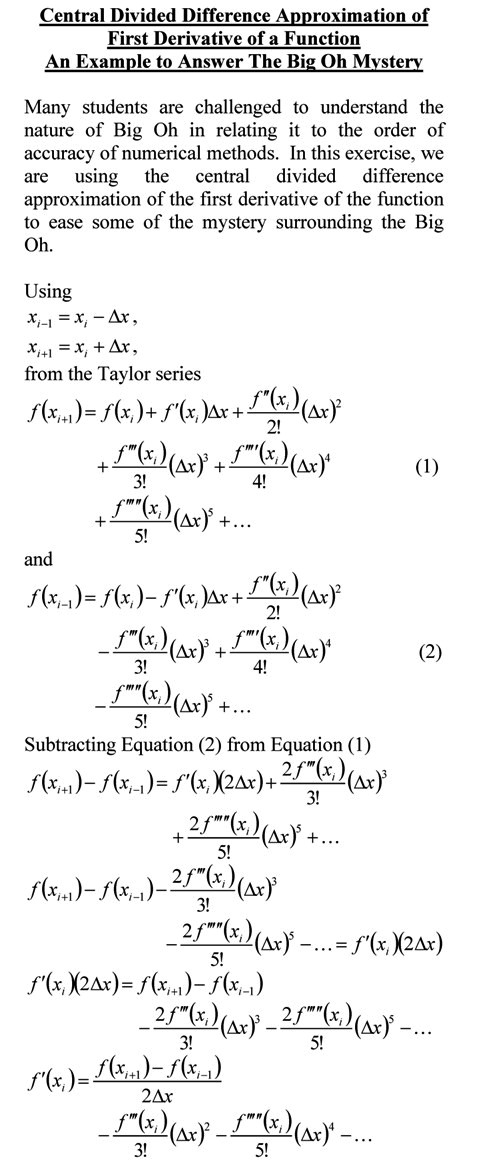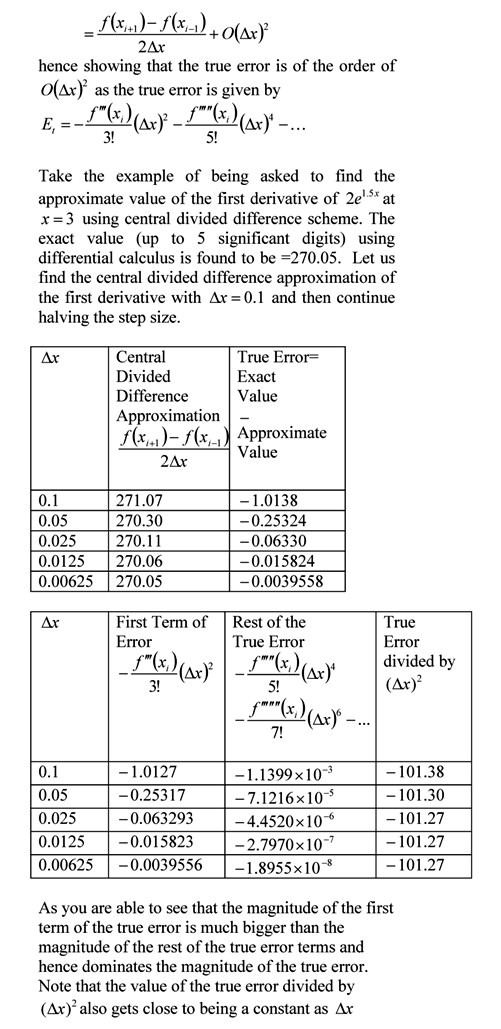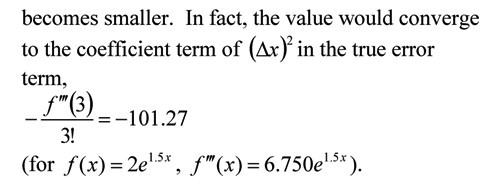## Making sense of the Big Oh!

Many students are challenged to understand the nature of Big Oh in relating it to the order of accuracy of numerical methods.  In this exercise, we are using the central divided difference approximation of the first derivative of the function to ease some of the mystery surrounding the Big Oh.You can visit the above example by opening a pdf file.

This post is brought to you by Holistic Numerical Methods: Numerical Methods for the STEM undergraduate at http://nm.MathForCollege.com, the textbook on Numerical Methods with Applications available from the lulu storefront, the textbook on Introduction to Programming Concepts Using MATLAB, and the YouTube video lectures available at http://nm.MathForCollege.com/videos.  Subscribe to the blog via a reader or email to stay updated with this blog. Let the information follow you.

## Using Taylor polynomial to approximately solve an ordinary differential equation

Taylor polynomial is an essential concept in understanding numerical methods. Examples abound and include finding accuracy of divided difference approximation of derivatives and forming the basis for Romberg method of numerical integration.

In this example, we are given an ordinary differential equation and we use the Taylor polynomial to approximately solve the ODE for the value of the dependent variable at a particular value of the independent variable. As a homework assignment, do the following.
1) compare the approximate solution with the exact one, and
2) get another approximate solution by using a third order Taylor polynomial.